Select Page
MATHESIS :: Essential
• Articles coming soon
• Articles coming soon
• Articles coming soon
• Articles coming soon
• Articles coming soon
• Articles coming soon

# Peano’s Arithmetic

As a reminder, here are Peano’s axioms, which concern the successor function, which intuitively “adds” to each natural integer.
These axioms allow us to reconstruct the whole arithmetic structure of the set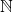of natural integers, and from naive set theory, all natural mathematical objects and structures.

Axiom 1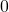is not the successor of any natural number. In other words, there is no natural number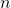such that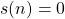.

This axiom says in particular that the function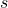is not surjective, since the numberhas no antecedent by.

Axiom 2

If two natural numbers have the same successor, then they are equal. In other words, for all natural numbers, if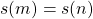then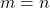.

We can reformulate this axiom by saying that the successor application is injective. It thus defines a bijection fromonto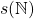. We recognise here the characterisation of an infinite set.

Proposition 1

The setof natural numbers is infinite.

Axiom 3 [Principle of induction (or recursion)]

If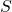is a subset ofsuch that:

1.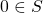2. for all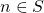,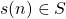(“induction step”),

then we have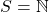.

This principle expresses intuitively that the setis entirely \og browsed” if we enumerate it starting fromand add each successive natural number, indefinitely.

We can deduce the determination of the image of:

Proposition 2

If we admit the induction principle, then the image ofis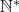, the set of non-zero natural numbers.

Demonstration

Letbe the set. If we show that, then any non-zero natural number is in the image of, so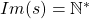. By definition, we have, and suppose thatis a natural number, and that. By definition, the natural integer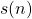is in! By the induction principle, the setis the whole set, and the proposition is proved.